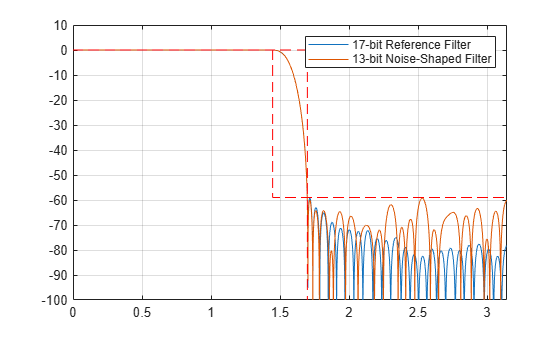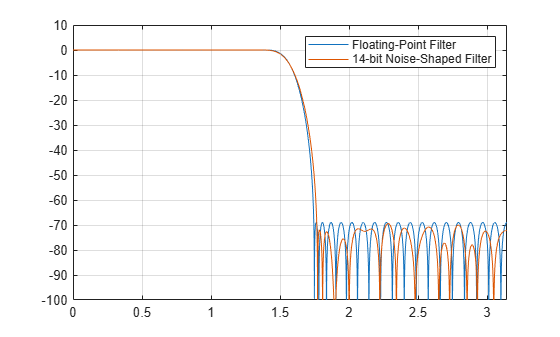# Optimized Fixed-Point FIR Filters

This example shows how to optimize fixed-point FIR filters. The optimization can refer to the characteristics of the filter response such as the stopband attenuation or the number of bits required to achieve a particular specification. This functionality is particularly useful for users targeting hardware that have a number of configurable coefficients of a specific wordlength and/or in cases typically found on ASICs and FPGAs where there is a large design space to explore. A hardware designer can usually trade off more coefficients for less bits or vice-versa to optimize for different ASICs or FPGAs.

This example illustrates various techniques based on the noise shaping procedure that yield optimized fixed-point FIR filter coefficients. The example shows how to:

• minimize coefficients wordlength,

• constrain coefficients wordlength,

• maximize stopband attenuation.

### Theoretical Background

The noise shaping algorithm essentially moves the quantization noise out of a critical frequency band (usually the stopband) of a fixed-point FIR filter at the expense of increasing it in other bands. The block diagram below illustrates the process of noise shaping. Essentially, the filter coefficients are passed through a system that resembles a digital filter, but with a quantizer in the middle. The system is computing the quantization error for each coefficient, then passing the error through a simple IIR highpass filter defined by the b1, b2 and b3 coefficients. The 'round' block rounds the input to the nearest quantized value. After this, the quantized value is subtracted from the original floating point value. The values of the initial state in each delay block can be set to random noise between -LSB and +LSB.The output of the system is the new, quantized and noise shaped filter coefficients. By repeating this procedure many times with different random initial states in the delay blocks, different filters can be produced.

### Minimize Coefficients Wordlength

To begin with, we want to determine the minimum wordlength fixed-point FIR filter that meets a single-stage or multistage design specification. We take the example of a halfband filter with a normalized transition width of .08 and a stopband attenuation of 59 dB. A Kaiser window design yields 91 double-precision floating-point coefficients to meet the specifications.

```TW = .08; % Transition Width Astop = 59; % Stopband Attenuation (dB) f = fdesign.halfband('TW,Ast', TW, Astop); Hd = design(f, 'kaiserwin');```

To establish a baseline, we quantize the filter by setting its '`Arithmetic`' property to 'fixed' and by iterating on the coefficients' wordlength until the minimum value that meets the specifications is found. Alternatively, we can use the `minimizecoeffwl`() to speed up the process. The baseline fixed-point filter contains 91 17-bit coefficients.

```Hqbase = minimizecoeffwl(Hd,... MatchRefFilter=true, NoiseShaping=false, ... Astoptol=0); % 91 17-bit coefficients, Astop = 59.1 dB```

The 17-bit wordlength is unappealing for many hardware targets. In certain situations we may be able to compromise by using only 16-bit coefficients. Notice however that the original specification is no longer strictly met since the maximum stopband attenuation of the filter is only 58.8 dB instead of the 59 dB desired.

```Hq1 = copy(Hqbase); Hq1.CoeffWordLength = 16; % 91 16-bit coefficients, Astop = 58.8 dB m1 = measure(Hq1) ```
```m1 = Sample Rate : N/A (normalized frequency) Passband Edge : 0.46 3-dB Point : 0.49074 6-dB Point : 0.5 Stopband Edge : 0.54 Passband Ripple : 0.017157 dB Stopband Atten. : 58.8741 dB Transition Width : 0.08 ```

Alternatively, we can set a tolerance to control the stopband error that is acceptable. For example, with a stopband tolerance of .15 dB we can save 3 bits and get a filter with 91 14-bit coefficients.

```Hq2 = minimizecoeffwl(Hd,... MatchRefFilter=true, NoiseShaping=false, ... Astoptol=.15); % 91 14-bit coefficients, Astop = 58.8 dB```

The saving in coefficients wordlength comes at the price of the fixed-point design no longer meeting the specifications. Tolerances can vary from one application to another but this strategy may have limited appeal in many situations. We can use another degree of freedom by relaxing the 'MatchRefFilter' constraint. By setting the '`MatchRefFilter`' property to false, we no longer try to match the filter order (for minimum-order designs) or the filter transition width (for fixed order designs) of Hd. Allowing a re-design of the intermediate floating-point filter results in fixed-point filter that meets the specifications with 93 13-bit coefficients. Compared to the reference fixed-point designs, we saved 4 bits but ended up with 2 extra (1 non zero) coefficients.

```Hq3 = minimizecoeffwl(Hd,... MatchRefFilter=false, NoiseShaping=false); % 93 13-bit coefficients```

A better solution yet is to use noise shaping to maximize the stopband attenuation of the quantized filter. The noise shaping procedure is stochastic. You may want to experiment with the '`NTrials`' option and/or to initialize RAND in order to reproduce the results below. Because '`MatchRefFilter`' is false by default and '`NoiseShaping`' is true, we can omit them. The optimized fixed-point filter meets the specifications with 91 13-bit coefficients. This represents a saving of 4 bits over the reference fixed-point design with the same number of coefficients.

```Hq4 = minimizecoeffwl(Hd, Ntrials=10); % 91 13-bit coefficients hfvt = fvtool(Hqbase,Hq4, ShowReference='off',Color='white'); legend(hfvt, '17-bit Reference Filter', '13-bit Noise-Shaped Filter');```As a trade-off of the noise being shaped out of the stopband, the passband ripple of the noise-shaped filter increases slightly, which is usually not a problem. Also note that there is no simple relationship of passband ripple to frequency after the noise-shaping is applied.

`zoom(hfvt,[0 0.5060 -0.0109 0.0109])`### Constrain Coefficients Wordlength

We have seen previously how we can trade-off more coefficients (or a larger transition width for designs with a fixed filter order) for a smaller coefficients wordlength by setting the '`MatchRefFilter`' parameter of the `minimizecoeffwl`() method to 'false'. We now show how we can further control this trade-off by relaxing this constraint.

```fm = fdesign.lowpass('Fp,Fst,Ap,Ast',0.1,0.12,1,70); Hm = design(fm);```

We first match the order of the floating-point design and obtain a noise-shaped fixed-point filter that meets the specifications with 237 coefficients of a 20-bits wordlength.

```Hmref = minimizecoeffwl(Hm, MatchRefFilter=true); disp(Hmref)```
``` FilterStructure: 'Direct-Form FIR' Arithmetic: 'fixed' Numerator: [1x237 double] PersistentMemory: false CoeffWordLength: 20 CoeffAutoScale: true Signed: true InputWordLength: 16 InputFracLength: 15 FilterInternals: 'FullPrecision' ```

By relaxing the matching requirement (thus letting the filter order increase), we get 247 coefficients (a modest increase over the previous case) with a reduced wordlength of 15-bits.

```Hq5 = minimizecoeffwl(Hm, MatchRefFilter=false); disp(Hq5);```
``` FilterStructure: 'Direct-Form FIR' Arithmetic: 'fixed' Numerator: [1x247 double] PersistentMemory: false CoeffWordLength: 15 CoeffAutoScale: true Signed: true InputWordLength: 16 InputFracLength: 15 FilterInternals: 'FullPrecision' ```

For better control of the final wordlengths, use the `constraincoeffwl`() method. For example, constrain the design to 16 bits coefficients.

```WL = 16; Hqc = constraincoeffwl(Hm,WL); disp(Hqc)```
``` FilterStructure: 'Direct-Form FIR' Arithmetic: 'fixed' Numerator: [1x243 double] PersistentMemory: false CoeffWordLength: 16 CoeffAutoScale: true Signed: true InputWordLength: 16 InputFracLength: 15 FilterInternals: 'FullPrecision' ```

### Maximize Stopband Attenuation

When designing for shelf filtering engines (ASSPs) that have a number of configurable coefficients of a specific wordlength, it is desirable to maximize the stopband attenuation of a filter with a given order and a constrained wordlength. In the next example, we wish to obtain 69 dB of stopband attenuation with a 70th order halfband decimator while using 14 bits to represent the coefficients.

```fh = fdesign.halfband('N,Ast',70,69); Hb1 = design(fh, 'equiripple');```

If we simply quantize the filter with 14-bit coefficients, we get only 62.7 dB of attenuation.

```Hb1.Arithmetic= 'fixed'; Hb1.CoeffWordLength = 14; mb1 = measure(Hb1)```
```mb1 = Sample Rate : N/A (normalized frequency) Passband Edge : 0.44518 3-dB Point : 0.48816 6-dB Point : 0.5 Stopband Edge : 0.55482 Passband Ripple : 0.010552 dB Stopband Atten. : 62.7048 dB Transition Width : 0.10963 ```

By shaping the noise out of the stopband we can improve the attenuation by almost 1.5 dB to 64.18 dB but we still cannot meet the specifications.

```Hbq1 = maximizestopband(Hb1, 14); mq1 = measure(Hbq1)```
```mq1 = Sample Rate : N/A (normalized frequency) Passband Edge : 0.44562 3-dB Point : 0.48812 6-dB Point : 0.5 Stopband Edge : 0.55367 Passband Ripple : 0.010959 dB Stopband Atten. : 63.8364 dB Transition Width : 0.10805 ```

The next step is to over design a floating-point filter with 80 dB of attenuation. We pay the price of the increased attenuation in the form of a larger transition width. The attenuation of the 14-bit non noise-shaped filter improved from 62.7 dB to 66.2 dB but is still not meeting the specifications.

```fh.Astop = 80; Hb2 = design(fh,'equiripple'); Hb2.Arithmetic= 'fixed'; Hb2.CoeffWordLength = 14; mb2 = measure(Hb2)```
```mb2 = Sample Rate : N/A (normalized frequency) Passband Edge : 0.43464 3-dB Point : 0.48704 6-dB Point : 0.5 Stopband Edge : 0.56536 Passband Ripple : 0.0076847 dB Stopband Atten. : 66.2266 dB Transition Width : 0.13073 ```

The noise shaping technique gives us a filter that finally meets the specifications by improving the stopband attenuation by more than 3 dB, from 66.2 dB to 69.4 dB.

```Hbq2 = maximizestopband(Hb2,14); mq2 = measure(Hbq2)```
```mq2 = Sample Rate : N/A (normalized frequency) Passband Edge : 0.43584 3-dB Point : 0.4871 6-dB Point : 0.5 Stopband Edge : 0.56287 Passband Ripple : 0.0053253 dB Stopband Atten. : 69.4039 dB Transition Width : 0.12703 ```

The transition width of the fixed-point filter is increased compared to the floating-point design. This is the price to pay to get 69 dB of attenuation with only 14-bit coefficients as it would take 24-bit coefficients to match both the transition width and the stopband attenuation of the floating-point design.

```close(hfvt); hfvt = fvtool(reffilter(Hb1),Hbq2, ShowReference='off', Color='white'); legend(hfvt,'Floating-Point Filter','14-bit Noise-Shaped Filter');````close(hfvt)`

### Summary

We have seen how the noise shaping technique can be used to minimize the coefficients wordlength of a single stage or multistage FIR fixed-point filter or how it can be used to maximize the stopband attenuation instead. We have also seen how bits can be traded for more coefficients in case of minimum order designs or for a larger transition width in case of designs with a fixed order.

 Jens Jorgen Nielsen, Design of Linear-Phase Direct-Form FIR Digital Filters with Quantized Coefficients Using Error Spectrum Shaping Techniques, IEEE® Transactions on Acoustics, Speech, and Signal Processing, Vol. 37, No. 7, July 1989, pp. 1020--1026.

 Alan V. Oppenheim and Ronald W. Schafer, Discrete-Time Signal Processing, 2nd edition, Prentice Hall, 1999, ISBN 0-13-754920-2.